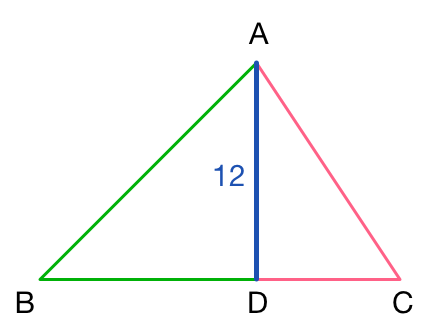# Back To Back Right Triangles

Geometry Level 2In the above figure, $ABC$ is a right triangle with height $AD$, resulting in Pythagorean triangles $ABD$ and $ACD$. If $AD = 12$, what is the length of $BC$?

Clarification: A Pythagorean Triangle is a right triangle with sides of integer length.

×